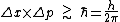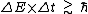# uncertainty principle

(redirected from Heisenberg uncertainty principal)
Also found in: Dictionary, Thesaurus.
Related to Heisenberg uncertainty principal: Pauli exclusion principle

## uncertainty principle,

physical principle, enunciated by Werner Heisenberg in 1927, that places an absolute, theoretical limit on the combined accuracy of certain pairs of simultaneous, related measurements. The accuracy of a measurement is given by the uncertainty in the result; if the measurement is exact, the uncertainty is zero. According to the uncertainty principle, the mathematical product of the combined uncertainties of simultaneous measurements of position and momentum in a given direction cannot be less than Planck's constantPlanck's constant
, fundamental constant of the quantum theory. It is represented by the letter h and has a value of 6.62607 × 10−34 joule-second. The combination h/2π, denoted by h (called "h-bar"), occurs frequently.
h divided by 4π. The principle also limits the accuracies of simultaneous measurements of energy and of the time required to make the energy measurement. The value of Planck's constant is extremely small, so that the effect of the limitations imposed by the uncertainty principle are not noticeable on the large scale of ordinary measurements; however, on the scale of atoms and elementary particles the effect of the uncertainty principle is very important. Because of the uncertainties existing at this level, a picture of the submicroscopic world emerges as one of statistical probabilities rather than measurable certainties. On the large scale it is still possible to speak of causality in a framework described in terms of space and time; on the atomic scale this is not possible. Such a description would require exact measurements of such quantities as position, speed, energy, and time, and these quantities cannot be measured exactly because of the uncertainty principle. It does not limit the accuracy of single measurements, of nonsimultaneous measurements, or of simultaneous measurements of pairs of quantities other than those specifically restricted by the principle. Even so, its restrictions are sufficient to prevent scientists from being able to make absolute predictions about future states of the system being studied. The uncertainty principle has been elevated by some thinkers to the status of a philosophical principle, called the principle of indeterminacy, which has been taken by some to limit causality in general. See quantum theoryquantum theory,
modern physical theory concerned with the emission and absorption of energy by matter and with the motion of material particles; the quantum theory and the theory of relativity together form the theoretical basis of modern physics.
.

### Bibliography

See W. Heisenberg, The Physical Principles of the Quantum Theory (tr. 1949); D. Lindley, Uncertainty (2007).

## Uncertainty principle

A fundamental principle of quantum mechanics, which asserts that it is not possible to know both the position and momentum of an object with arbitrary accuracy. This contrasts with classical physics, where the position and momentum of an object can both be known exactly. In quantum mechanics, this is no longer possible, even in principle. More precisely, the indeterminacy or uncertainty principle, derived by W. Heisenberg, asserts that the product of Δx and Δp—measures of indeterminacy of a coordinate and of momentum along that coordinate—must satisfy inequality (1).

(1)The Planck constant, h &simeq; 6.63 × 10-34 joule-second, is very small, which makes inequality (1) unimportant for the measurements that are carried out in everyday life. Nevertheless, the consequences of the inequality are critically important for the interactions between the elementary constituents of matter, and are reflected in many of the properties of matter that are ordinarily taken for granted. For example, the density of solids and liquids is set to a large degree by the uncertainty principle, because the sizes of atoms are determined with decisive help of inequality (1).

In classical physics, simultaneous knowledge of position and momentum can be used to predict the future trajectory of a particle. Quantum indeterminacy and the limitations it imposes force such classical notions of causality to be abandoned.

Another well-known example of indeterminacy involves energy and time, as given by inequality (2).

(2)Physically, its origins are somewhat different from those of inequality (1). Inequality (2) relates, for example, lifetimes of unstable states with the widths of their lines. See Linewidth

In quantum physics, relations similar to inequalities (1) and (2) hold for pairs of many other quantities. They demonstrate that the acquisition of the information about a quantum object cannot be usually achieved without altering its state. Much of the strangeness of quantum physics can be traced to this impossibility of separating the information about the state from the state itself. See Quantum mechanics

McGraw-Hill Concise Encyclopedia of Physics. © 2002 by The McGraw-Hill Companies, Inc.
The following article is from The Great Soviet Encyclopedia (1979). It might be outdated or ideologically biased.

## Uncertainty Principle

(also indeterminacy principle), a fundamental proposition of quantum theory that asserts that no physical system can exist in states in which the coordinates of its center of mass and its momentum simultaneously assume completely determined, exact values. A quantitative statement of the uncertainty principle is the following: if ∆x is the uncertainty in the value of the x-coordinate and ∆px is the uncertainty in the component of the momentum along the x-axis, then the product of these uncertainties should have a magnitude not less than that of Planck’s constant ћ. Similar inequalities must be satisfied for any pair of canonically conjugate variables, for example, (1) the y-coordinate and the component of the momentum py along the y-axis or (2) the z-coordinate and the component of the momentum pZ. If by the uncertainties in the coordinate and the momentum we mean the root-mean-square deviations of these physical quantities from their average values, then the uncertainty relation assumes the form

∆px∆x ≥ ℏ/2, ∆py∆y ≥ ℏ/2, ∆pzz ≥ h̷/2

Because ħ is so small in comparison with macroscopic quantities of the same dimensionality, namely, action, the uncertainty relations are significant primarily for phenomena on the atomic (or smaller) scale and are not apparent in interactions of macroscopic bodies.

It follows from the uncertainty relation that the more precisely one of the quantities in the inequality is determined, the less precise is the determination of the value of the other. No experiment can simultaneously yield precise measurements of both of these dynamic variables; in this case, the uncertainty in the measurements is related not to imperfections in the experimental techniques but to the intrinsic properties of matter.

The uncertainty principle, which was discovered by W. Heisenberg in 1927, was an important step in setting up the quantum mechanics and elucidating the principles that govern subatomic phenomena. A distinctive feature of microscopic objects is the particle-wave duality of their nature. The state of a particle is fully determined by a wave function. The particle can be detected at any point in space at which the wave function is nonzero. Therefore, results of experiments designed to determine a coordinate, for example, are probabilistic in nature. This means that, in general, in carrying out a series of identical experiments on identical systems, a different value is obtained in each experiment. However, certain values will be more probable than others, that is, they will occur more often. The relative frequency of occurrence of the different values of the coordinate is proportional to the square of the absolute value of the wave function at the corresponding points in space. Therefore, values of the coordinate that lie near the maximum of the wave function will be obtained most often. If the maximum is sharp (if the wave function represents a narrow wave packet), then the particle is located “for the most part” near this maximum. Nonetheless, some spread in the values of the coordinate and some uncertainty (of the order of the half-width of the maximum) are unavoidable. The same conclusion also applies to the measurement of momentum.

Thus, the concepts of coordinate and momentum in the classical sense cannot be applied to microscopic objects. In using these quantities to describe a microscopic system, quantum corrections must be introduced into their interpretation. The uncertainty relation is such a correction.

The uncertainty relation has a somewhat different meaning for the energy E and the time t, ∆E∆t≳ ℏ. If the system is in a stationary state, that is, a state that does not change in the absence of external forces, then it follows from the uncertainty relation that the energy of the system in this state can be measured only with an accuracy not better than ℏ/∆t, where ∆t is the duration of the measurement process. This result is due to the interaction of the system with the measuring device; in this case, the uncertainty relation means that the interaction energy between the measuring device and the system under study can be taken into account only with an accuracy not better than ℏ/∆t. (In the limiting case of instantaneous measurement, the energy exchange that arises becomes completely indeterminate.) The relation ∆E∆t ≳ ℏ also holds if ∆E is understood to mean the uncertainty in the value of the energy of a nonstationary state of a closed system, and ∆t is the characteristic time required for the average values of the physical quantities in the system to change significantly.

The uncertainty relation for energy and time leads to important conclusions concerning the excited states of atoms, molecules, and nuclei. Such states are unstable, and it follows from the uncertainty relation that the energies of the excited levels cannot be strictly determined, that is, the levels have a certain width (the natural width of the level). If ∆t is the average lifetime of the excited state, then the width of the corresponding energy level (the uncertainty of the energy of the state) is ∆E ≈ ℏ/∆t. The alpha decay of a radioactive nucleus is another example: the energy spread ∆E of the alpha particles that are emitted is related to the lifetime τ of such a nucleus by the equation ∆E ≈ ℏ/τ.

### REFERENCES

Heisenberg, W., E. Schrödinger, and P. Dirac. Sovremennaia kvantovaia mekhanika. Moscow-Leningrad, 1934. (Translated from English.)
Dirac, P. Printsipy kvantovoi mekhaniki. Moscow, 1960. (Translated from English.)
Blokhintsev, D. I. Osnovy kvantovoi mekhaniki, 3rd ed. Moscow, 1961.
Mandel’shtam, L. I., and I. E. Tamm. “Sootnoshenie neopredelennosti energiia-vremia ν nereliativistskoi kvantovoi mekhanike.” In L. I. Mandel’shtam, Poln. sobr. trudov, vol. 2. Moscow-Leningrad, 1947. Page 306.
Krylov, N. S., and V. A. Fok. “O dvukh osnovnykh tolkovaniiakh sootnosheniia neopredelennosti dlia energii i vremeni.” Zhurnal eksperimental’noi i teoreticheskoi fiziki, 1947, vol. 17, issue 2, p. 93.

O. I. ZAV’IALOV